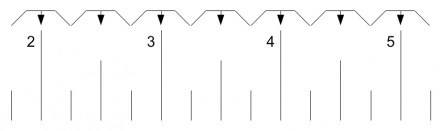# How Do You Measure To The Nearest 1/2 Inch?

## 2 Answers

Devon Smith answered
If the measurement doesn't reach the 1/2 inch mark between inch markers, round down to the whole inch. If the measurement is at or above 1/2 inch, use the half inch mark in your measurment.
thanked the writer.
Oddman answered
Locate the nearest inch or half-inch mark and use that to express your measurement.

If the measurement is exactly on the 1/4 inch mark, you round up to the nearest 1/2 inch. Likewise, if the measurement is exactly on the 3/4 inch mark, you round up to the nearest whole inch.

In this illustration, each angle bracket and arrow shows a range and the nearest half-inch to which it will be rounded. Each quarter gets rounded up to the nearest half/whole.thanked the writer.

## Answer Question